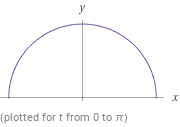Search IntMath
Close

450+ Math Lessons written by Math Professors and Teachers

5 Million+ Students Helped Each Year

1200+ Articles Written by Math Educators and Enthusiasts

Simplifying and Teaching Math for Over 23 Years

# Understanding Semicircles in Geometry## What Is a Semicircle?

A semicircle is a geometric shape with one curved side and two straight sides. It is a half of a circle, hence the name. A semicircle is formed by taking a circular arc and connecting its endpoints to form a straight line. The curved side of a semicircle is called a semicircular arc, and the straight sides are called the diameter of the semicircle.

## How Is the Radius of a Semicircle Measured?

The radius of a semicircle is the distance from the center of the semicircle to any point on the semicircular arc. The diameter of a semicircle is the length of the straight line that connects the two endpoints of the semicircular arc. The radius of a semicircle is always equal to half of its diameter.

## How Is the Area of a Semicircle Calculated?

The area of a semicircle can be calculated using the following formula:

This formula is derived from the area of a circle formula, which is p x (Radius)2. Since a semicircle is half of a circle, the area formula for a semicircle is half of the area formula for a circle.

## What Are Some Applications of Semicircles in Geometry?

Semicircles are commonly used in a variety of geometric problems. For example, semicircles can be used to calculate the area of a sector of a circle, or the area of an isosceles triangle. They can also be used to calculate the circumference of a circle, or the length of an arc.

## Practice Problems

1. Find the area of a semicircle with a radius of 4 cm.

Answer: Area = p x (4 cm)2 = 12.57 cm2

2. Find the circumference of a semicircle with a diameter of 12 cm.

Answer: Circumference = p x (6 cm) = 18.85 cm

3. Find the radius of a semicircle with an area of 25 cm2.

4. Find the diameter of a semicircle with a circumference of 24 cm.

Answer: Diameter = (24 cm) / p = 7.65 cm

5. Find the area of a sector of a circle with a radius of 8 cm and an arc length of 24 cm.

Answer: Area = (24 cm x (8 cm)2) / (2 x p) = 38.48 cm2

## Summary

In this article, we have discussed semicircles in geometry. We learned that a semicircle is a geometric shape with one curved side and two straight sides, and that the radius of a semicircle is always equal to half of its diameter. We also discussed the formula for calculating the area of a semicircle and the applications of semicircles in geometry. Finally, we have provided some practice problems to help you understand and apply the concepts discussed in this lesson.

## FAQ

### What is semicircle with example?

A semicircle is a two-dimensional shape that is created by drawing a line from one end of a circle to the other. It is half of the circle and looks like half of an oval. For example, the face of a clock is a semicircle.

### What is a semicircle called?

A semicircle is also known as a hemicycle, semi-circle, or semicircle.

## Problem SolverThis tool combines the power of mathematical computation engine that excels at solving mathematical formulas with the power of GPT large language models to parse and generate natural language. This creates math problem solver thats more accurate than ChatGPT, more flexible than a calculator, and faster answers than a human tutor. Learn More.